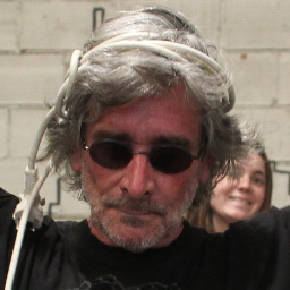Bibliothèque de traitements d'images en virgule flottante. http://la.buvette.org/photos/cumul/
You can not select more than 25 topics Topics must start with a letter or number, can include dashes ('-') and can be up to 35 characters long.

#### 44 lines 990 B Raw Normal View History Unescape Escape4 years ago ```#--------------------------------------------------------------- ``` ``` ```3 years ago ```COPT = -Wall -fpic -g -no-pie -DDEBUG_LEVEL=0 ```4 years ago ```DEPS = ../floatimg.h Makefile ```3 years ago ```OBJS = fimg-png.o fimg-tiff.o misc-plots.o filtrage.o utils.o \ ```3 years ago ``` fimg-libpnm.o rampes.o sfx0.o geometry.o ```3 years ago ``` ``` ```#--------------------------------------------------------------- ``` ``` ``` ```t: t.c \$(DEPS) ../libfloatimg.a ```3 years ago ``` gcc \$(COPT) \$< ../libfloatimg.a -lnetpbm -lpnglite -lz -lm -o \$@ ```4 years ago ``` ``` ```#--------------------------------------------------------------- ``` ``` ``` ```../libfloatimg.a: \$(OBJS) ``` ``` \$(AR) r \$@ \$? ``` ``` ``` ```fimg-png.o: fimg-png.c \$(DEPS) ``` ``` gcc \$(COPT) -c \$< ``` ``` ``` ```fimg-tiff.o: fimg-tiff.c \$(DEPS) ``` ``` gcc \$(COPT) -c \$< ``` ``` ```3 years ago ```fimg-libpnm.o: fimg-libpnm.c \$(DEPS) ``` ``` gcc \$(COPT) -c \$< ``` ``` ```4 years ago ```misc-plots.o: misc-plots.c \$(DEPS) ``` ``` gcc \$(COPT) -c \$< ``` ``` ``` ```filtrage.o: filtrage.c \$(DEPS) ``` ``` gcc \$(COPT) -c \$< ```3 years ago ``` ```3 years ago ```geometry.o: geometry.c \$(DEPS) ``` ``` gcc \$(COPT) -c \$< ``` ``` ```3 years ago ```sfx0.o: sfx0.c \$(DEPS) ``` ``` gcc \$(COPT) -c \$< ``` ``` ```3 years ago ```rampes.o: rampes.c \$(DEPS) ``` ``` gcc \$(COPT) -c \$< ``` ``` ```3 years ago ```utils.o: utils.c \$(DEPS) ``` ``` gcc \$(COPT) -c \$< ```